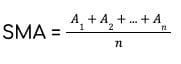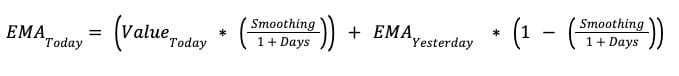Crypto Terms: Letter M

# What is Moving Average (MA)?

Moving Average (MA) MEANING:
Moving Average (MA) - a technical indicator used by marketers to forecast the direction of the trend of an asset.
Medium
2 minutes

Let's find out Moving Average (MA) meaning, definition in crypto, what is Moving Average (MA), and all other detailed facts.

Moving Average (MA) is a technical indicator that reacts to changes in financial market trends. Therefore, it is used by marketers to forecast the direction of the trend of an asset. The basic concept of MA is to take an asset's average closing price over an X amount of periods.

The MA graph usually consists of two lines (short-term MA and long-term MA). The gap between these lines indicates the amount of volume. If the gap is huge, it means that the level of volume is really high.

When the short-term MA line crosses the long-term MA line from below going up, it’s called a golden cross, and it signals a bullish trend (the price is increasing). However, when the short-term MA line crosses the long-term MA line from above going down, it’s called a death cross, and it means that there is a bearish trend in the price of an asset (the price is decreasing).

### Why Is Moving Average (MA) Used?

Marketers use the MA to evaluate an asset's support and resistance by analyzing its market movements. The MA depicts the price activity in a straightforward manner that can be utilized by investors to predict a probability of a bullish or bearish trend.

### What is the Best Moving Average (MA) Setting?

The best setting for Moving Average (MA) is:

• Short-term MA – 50 days
• Long-term MA – 200 days

## Types of Moving Averages (MAs)

Overall there are four types of MAs – Smoothed, Weighted, Exponential, and Simple (or Arithmetic). However, Simple Moving Average (SMA) and Exponential Moving Average (EMA) are the two most popular ones in the financial industry.

### Simple Moving Average (SMA)

The sum of all the data points in a given time period is divided by the total number of time periods to generate a Simple Moving Average (SMA).

The formula to calculate the SMA:Where:

n = total number of time periods

A = average in an ‘n’ period

### Exponential Moving Average (EMA)

The Exponential Moving Average (EMA) focuses on recent price data while also retaining previous price insights for traders to examine and make informed investment decisions. Therefore, traders prefer using EMA more than SMA.

The formula to calculate the EMA:Where:

EMA = Exponential Moving Average

Smoothing = 2 (increase it if you want the recent price observations to have a bigger impact on the EMA)

MA is one of the better indicators for traders, however, it’s best to use a combination of various indicators. Some of the other useful ones include the Aroon indicator, OBV, MACD, RSI, and the stochastic oscillator.